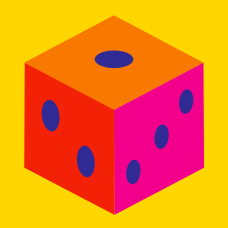Probability

Uniform Probability

A die is made from a regular icosahedron so that each side labelled by a number from $1$ through $10,$ with some labels appearing multiple times. If the the probability that it lands on the number $8$ is $\frac{1}{5},$ how many sides are labelled $8?$

Details and assumptions

A regular icosahedron has $20$ faces.

A dartboard is equally divided into fan-shaped sectors, each with a central angle of $20 ^\circ.$ If exactly one of the sectors is painted red and a dart has equal probability of hitting every position in the dartboard, then the probability that the dart hits the red sector can be expressed as $\frac{a}{b}$, where $a$ and $b$ are coprime positive integers. What is $a+b?$

Details and assumptions

Assume that the dart never misses the dartboard.

A dartboard is equally divided into fan-shaped sectors, each with a central angle of $6 ^\circ$, and exactly one of the sectors is painted red. If the dart has equal probability of hitting every position in the dartboard, what is the probability that it hit the red sector?

Details and assumptions

Assume that the dart never misses the dartboard.

Sophia rolls a fair standard 6-sided die with sides labeled $1$ through $6$. What is the probability that she rolls a $6$?

Details and assumptions

In a fair die, each side is equally likely to be rolled.

A magician randomly draws one card from a standard $52$ card deck. The probability that the card drawn is a heart or a diamond can be written as $\frac{a}{b}$, where $a$ and $b$ are positive, coprime integers. What is the value of $a+b$?

Details and assumptions

A standard deck of cards consists of 52 cards, formed by 13 ranks (1, 2, 3, 4, 5, 6, 7, 8, 9, 10, J, Q and K) of 4 suits (clubs, diamonds, hearts and spades).

×